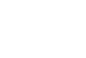[ML-Heavy] 建立 GAN 模型

G(z) 生成假的图片

[ML-Heavy] DCGAN

[ML-Heavy] 实现 DCGAN

### 第二步：快速生成假的图片

#### 从未知的概率分布中学习生成新的样本Yann LeCun 在回答中简单介绍了 GAN 的基本原理，也就是两个网络相互博弈的过程。

#### [ML-Heavy] 建立 GAN 模型

GANs 这个想法是 Ian Goodfellow 在其带有里程碑意义的论文“Generative Adversarial Nets” (GANs)发表在 2014 年的 Neural Information Processing Systems (NIPS) 会议上后开始火遍整个深度学习领域的。这个想法就是我们首先定义一个简单并众所周知的概率分布，并表示，在本文后面，我们用表示在[-1,1)（包含-1，但不包含1）范围的均匀分布。用表示从这个分布中采样，如是一个五维的，我们可以利用下面一行的 Python 代码来进行采样得到，这里用到 numpy这个库：

```z = np.random.uniform(-1, 1, 5)
array([ 0.77356483,  0.95258473, -0.18345086,  0.69224724, -0.34718733])```

```def G(z):
...
return imageSample
z = np.random.uniform(-1, 1, 5)
imageSample = G(z)```

https://github.com/vdumoulin/conv_arithmetic#### 采用 G(z) 生成假的图片#### [ML-Heavy] 训练 DCGAN#### 目前的 GAN 和 DCGAN 实现

https://github.com/tqchen/mxnet-gan

https://github.com/Newmu/dcgan_code

https://github.com/soumith/dcgan.torch

https://github.com/carpedm20/DCGAN-tensorflow

https://github.com/openai/improved-gan

https://github.com/mattya/chainer-DCGAN

https://github.com/jacobgil/keras-dcgan

#### [ML-Heavy] TensorFlow 实现 DCGAN

https://github.com/bamos/dcgan-completion.tensorflow

```def generator(self, z):
self.z_, self.h0_w, self.h0_b = linear(z, self.gf_dim*8*4*4,
'g_h0_lin', with_w=True)
self.h0 = tf.reshape(self.z_, [-1, 4, 4, self.gf_dim * 8])
h0 = tf.nn.relu(self.g_bn0(self.h0))
self.h1, self.h1_w, self.h1_b = conv2d_transpose(h0,
[self.batch_size, 8, 8, self.gf_dim*4], name='g_h1', with_w=True)
h1 = tf.nn.relu(self.g_bn1(self.h1))
h2, self.h2_w, self.h2_b = conv2d_transpose(h1,
[self.batch_size, 16, 16, self.gf_dim*2], name='g_h2', with_w=True)
h2 = tf.nn.relu(self.g_bn2(h2))
h3, self.h3_w, self.h3_b = conv2d_transpose(h2,
[self.batch_size, 32, 32, self.gf_dim*1], name='g_h3', with_w=True)
h3 = tf.nn.relu(self.g_bn3(h3))
h4, self.h4_w, self.h4_b = conv2d_transpose(h3,
[self.batch_size, 64, 64, 3], name='g_h4', with_w=True)
return tf.nn.tanh(h4)
def discriminator(self, image, reuse=False):
if reuse:
tf.get_variable_scope().reuse_variables()
h0 = lrelu(conv2d(image, self.df_dim, name='d_h0_conv'))
h1 = lrelu(self.d_bn1(conv2d(h0, self.df_dim*2, name='d_h1_conv')))
h2 = lrelu(self.d_bn2(conv2d(h1, self.df_dim*4, name='d_h2_conv')))
h3 = lrelu(self.d_bn3(conv2d(h2, self.df_dim*8, name='d_h3_conv')))
h4 = linear(tf.reshape(h3, [-1, 8192]), 1, 'd_h3_lin')
return tf.nn.sigmoid(h4), h4```

```self.G = self.generator(self.z)
self.D, self.D_logits = self.discriminator(self.images)
self.D_, self.D_logits_ = self.discriminator(self.G, reuse=True)```

```self.d_loss_real = tf.reduce_mean(
tf.nn.sigmoid_cross_entropy_with_logits(self.D_logits,
tf.ones_like(self.D)))
self.d_loss_fake = tf.reduce_mean(
tf.nn.sigmoid_cross_entropy_with_logits(self.D_logits_,
tf.zeros_like(self.D_)))
self.d_loss = self.d_loss_real + self.d_loss_fake
self.g_loss = tf.reduce_mean(
tf.nn.sigmoid_cross_entropy_with_logits(self.D_logits_,
tf.ones_like(self.D_)))```

```t_vars = tf.trainable_variables()
self.d_vars = [var for var in t_vars if 'd_' in var.name]
self.g_vars = [var for var in t_vars if 'g_' in var.name]```

```d_optim = tf.train.AdamOptimizer(config.learning_rate, beta1=config.beta1) \
.minimize(self.d_loss, var_list=self.d_vars)
.minimize(self.g_loss, var_list=self.g_vars)```

```for epoch in xrange(config.epoch):
...
for idx in xrange(0, batch_idxs):
batch_images = ...
batch_z = np.random.uniform(-1, 1, [config.batch_size, self.z_dim]) \
.astype(np.float32)
# Update D network
_, summary_str = self.sess.run([d_optim, self.d_sum],
feed_dict={ self.images: batch_images, self.z: batch_z })
# Update G network
_, summary_str = self.sess.run([g_optim, self.g_sum],
feed_dict={ self.z: batch_z })
# Run g_optim twice to make sure that d_loss does not go to zero
# (different from paper)
_, summary_str = self.sess.run([g_optim, self.g_sum],
feed_dict={ self.z: batch_z })
errD_fake = self.d_loss_fake.eval({self.z: batch_z})
errD_real = self.d_loss_real.eval({self.images: batch_images})
errG = self.g_loss.eval({self.z: batch_z})```

#### 在你的数据集上运行 DCGAN 模型

https://github.com/bamos/dcgan-completion.tensorflow

https://github.com/bamos/dcgan-completion.tensorflow

http://cmusatyalab.github.io/openface

```git clone https://github.com/cmusatyalab/openface.git
git clone https://github.com/bamos/dcgan-completion.tensorflow.git```

http://cmusatyalab.github.io/openface/setup/

```cd openface
pip2 install -r requirements.txt
python2 setup.py install
models/get-models.sh
cd ..```

MS-Celeb-1M–https://www.microsoft.com/en-us/research/project/msr-image-recognition-challenge-irc/

CelebA–http://mmlab.ie.cuhk.edu.hk/projects/CelebA.html

CASIA-WebFace–http://www.cbsr.ia.ac.cn/english/CASIA-WebFace-Database.html

FaceScrub–http://vintage.winklerbros.net/facescrub.html

LFW–http://vis-www.cs.umass.edu/lfw/

MegaFace–http://megaface.cs.washington.edu/

`./openface/util/align-dlib.py data/dcgan-completion.tensorflow/data/your-dataset/raw align innerEyesAndBottomLip data/dcgan-completion.tensorflow/data/your-dataset/aligned --size 64`

```cd dcgan-completion.tensorflow/data/your-dataset/aligned
find . -name '*.png' -exec mv {} . \;
find . -type d -empty -delete
cd ../../..```

`./train-dcgan.py --dataset ./data/your-dataset/aligned --epoch 20`

`samples` 文件夹中可以查看保存的由 G 生成的图片。这里作者是采用手上有的两个数据集 CASIA-WebFace 和 FaceScrub 进行训练，并在训练 14 个 epochs 后，生成的结果如下图所示：`tensorboard --logdir ./logs`